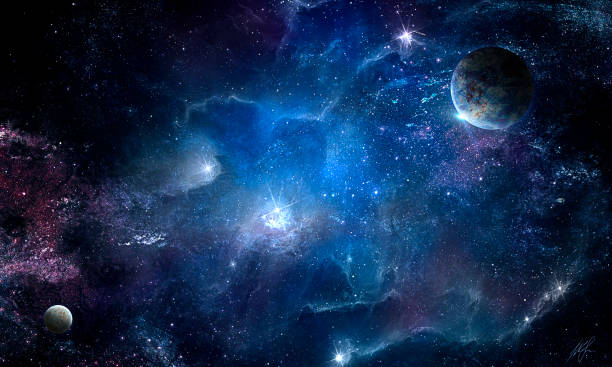Cosmic nebula and the shining stars, abstract space illustration

# The Models of The Universe

Posted on 382,860 viewsThe study of geometrical forms of Universe is based on topology. This exotic mathematics domain that everyone knows primarily through the Moebius strip shown at right, says for example that a cup with a handle and a torus have the same topology: both have a hole in their volume, space which changes the way the elements are connected, deformities that do not exist in a sphere for example.

This hole in hyperspace corresponds to the Euler number which is 1. In the case of a topological space without holes, which is more consistent with our universe, the Euler number = 0.

Topology is only interested in connections, and does not care of the size, shape, or curvature of the objects (in practice, the effect of gravity for example).

Topology may explain what happens to the space into a singularity and if the space-time regions are interconnected or not. We will discuss these possibilities when we discuss quantum cosmology.

Above all, Jean-Claude Pecker , honorary professor at the College de France, reminds us not to confuse the geometry of three-dimensional universe (x, y, z), and that of space-time (x, y, z, t).

In topology, the curvature of the space is defined as being equal to the product of the inverse of the radius of curvature of 2 main sections drawn on its surface. If such a sphere is cut transversely, we get two arcs of the same curvature. The curvature is called positive. If we cut a hyperbolic surface transversely to its axis, the two circular arcs have curvatures in opposite directions. The curvature is called negative.

The curvature is therefore measured directly on the surface and does not depend on the choice of coordinates. They say it is entirely determined by the metric.By studying topology, Friedmann discovered that a field of empty universe was not flat as we previously thought, but had a negative curvature, while the injection of material gave him a positive curvature. Friedmann discovered the following model:

• The Euclidean, three-dimensional model, applies to all forms of space respecting the laws of classical geometry, parallel lines infinitely never meeting. The area of ​​a circle (disc) is πr2, its volume being 4/3πr3. The universe as we know it may be represented by an infinite two-dimensional surface. In theory, the Euclidean model contains 10 infinite variants that extend to infinity and 8 finite, the cylindrical space, and the torus.
• The spherical model, symbolically represented by a sphere in which the postulates of Euclidean geometry no longer apply. The space is curved and closes on itself. Two parallel lines meeting at a distance determined by the radius of curvature, the area of ​​a circle is less than πr2, its volume is less than 4/3πr3. This geometric model contains an infinite number of finite forms of space without limits. In this model, our universe could be limited while having no border.
• The hyperbolic model (represented by a saddle without a center), in which the laws of Euclidean geometry no longer apply either. Understand what that means: when the distance doubles on a surface of positive curvature, the total surface remains below the square of the radius and vice versa on a hyperbolic negative surface! This is why, laid flat, the surface of a sphere has a recess and a negative surface has creases. The area of ​​a circle of a hyperbolic surface becomes higher than πr2 and its volume is greater than 4/3πr3. The universe is infinite. Whether it is open or closed, the hyperbolic model also includes an infinite number of variations.

As we will detail a little further, in the three models of the Universe, the radius of curvature of space, R, varies over time. This leads to two changes: if it grows, the universe is expanding, if it decreases, it is contracting.But these concepts are ambiguous. A hyperbolic or spherical three-dimensional universe may be constantly expanding, consider the famous image of the balloon that inflates. But at four dimensions, the same world can do what it wants, according to the change in radius with time! … The shape of the area, at 3 dimensions, depends on the distribution of the material. Given this ambiguity between spatial and temporal finitude, most astronomers have stopped … and prefer not to use these concepts.

In practice, if we can determine the area or volume of a region of space, we will be able to determine whether the universe is flat, open, or closed (Euclidean, hyperbolic, or spherical). However, this thesis is physically unprovable because it is physically impossible to measure the space of millions, even billions of light years. It is through the amount of matter it contains that we can determine its density, its volume, and predict its evolution. This determination presents many problems and sometimes leads to paradoxes. Only the scaffolding of new mathematical and physical hypotheses solve these dilemmas.

## 12 thoughts on “The Models of The Universe”

1.jay says:

g

2.jioj says:

jio

This site uses Akismet to reduce spam. Learn how your comment data is processed.